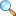Chapter 04: System of Linear Equations

Notes of the book Mathematical Method written by S.M. Yusuf, A. Majeed and M. Amin, published by Ilmi Kitab Khana, Lahore - PAKISTAN.

The difficulty level of this chapter is low. Most of the questions involve calculations. This chapter is wide range of applications in Linear Algebra and Operations Research. In many universities teachers include this chapter in the syllabus of Linear Algebra and Operations Research for BS students of mathematics and other subjects.

Contents and summary

• Preliminaries
• Equivalent equations
• Gaussian elimination method
• Gauss-Jordan elimination method
• Consistency criterion
• Network flow problems

• Exercise 4.1 |View online | Download PDF (0.99MB)